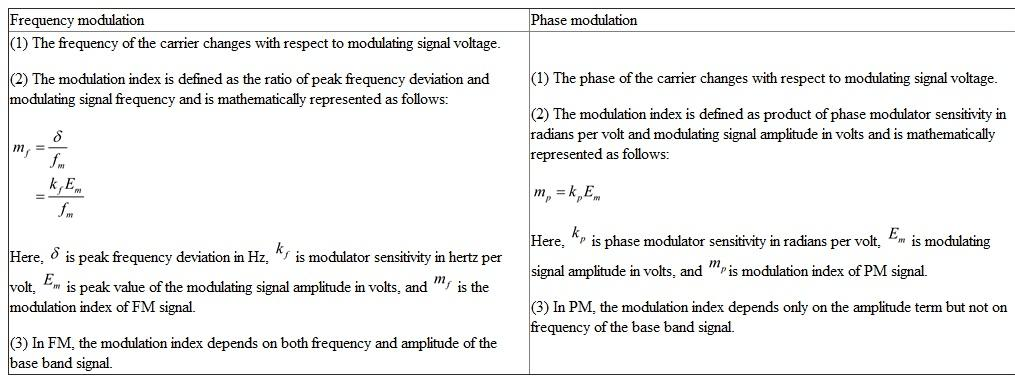# Quiz 4: Angle Modulation

Trade & Technology

The term angle modulation represents two types of modulations namely frequency modulation (FM) and phase modulation (PM). The frequency of carrier wave varies according to the amplitude of the modulating signal in frequency modulation. The phase of carrier wave varies with respect to modulating signal amplitude in phase modulation. Therefore, the term angle modulation is defined as both the frequency and phase of a carrier wave varies with respect to modulating signal amplitude. The main advantage of using either FM or PM is to achieve better signal to noise ratio compared to AM. The conversion form of FM to PM or the converse is easier one, since their mathematical equations are closely related to one another. The FM communication is used in daily life applications such as television, radio broadcasting, satellite communications, cellular systems and the PM communication is preferred in data communications. The usage of FM is more than PM. The FM transmitters in turn use PM as intermediate stage in some applications. The spectrum of single tone modulation by angle modulation technique represents infinite number of side bands. This spectrum consists of carrier and multiples of frequencies of modulating signal. The amplitude of the spectrum becomes less as the distance from the carrier frequency increases.

Phase Modulation: In this modulation method, the phase of carrier signal changes in accordance with the instantaneous amplitude of the modulating signal, where as amplitude and frequency of carrier signal remains constant. Frequency Modulation: In this modulation method, the frequency of carrier signal changes in accordance with the instantaneous amplitude of the modulating signal, where as amplitude and phase of carrier signal remains constant. Frequency and Phase for a sine wave is closely related to one another. To explain this further, Frequency is calculated as the derivative of the phase angle with reference to time or it is define as the rate of change of phase angle. Here, Phase angle is expressed in radians and frequency is expressed in radians per second. Therefore, whenever there is alteration in either of frequency or phase, there will be change in the other as well. The modulation index (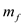) is given by following formula for angle modulated signal with sine wave modulation.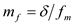Where,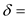Peak Phase Deviation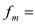Modulating Frequency So, the above equation depicts that modulation index is inversely proportional to modulating frequency and directly proportional to Peak phase deviation. Therefore, from above equation it is very clear, that when the frequency increases, the phase angle changes very quickly. That is, there will be greater increase in phase angle, because the change in frequency is also greater. And also phase deviation is inversely proportional to frequency of the modulating signal, that is, there is reduced change in phase angle when modulating frequency is increased. Hence, it can be concluded that phase is inversely proportional to frequency for a sine wave.

Comparison between frequency modulation (FM) and phase modulation (PM) in terms of modulation index: